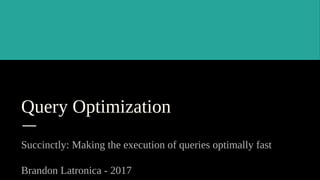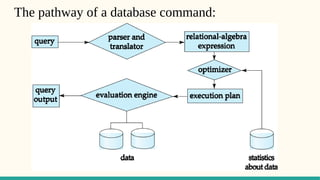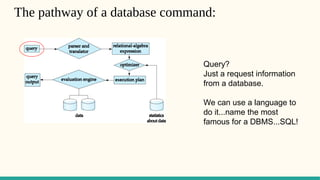Successfully reported this slideshow.

# Query Optimization - Brandon Latronica×

# Query Optimization - Brandon Latronica

Brandon Latronica did a nice presentation to introduce database query optimization.

Brandon Latronica did a nice presentation to introduce database query optimization.

### Query Optimization - Brandon Latronica

1. 1. Query Optimization Succinctly: Making the execution of queries optimally fast Brandon Latronica - 2017
2. 2. The pathway of a database command:
3. 3. The pathway of a database command: Query? Just a request information from a database. We can use a language to do it...name the most famous for a DBMS...SQL!
4. 4. The pathway of a database command: Parsing and translation: translate the query into its internal form. This is then translated into relational algebra. Parser checks syntax, verifies relations
5. 5. The pathway of a database command: Relational Algebra: The conversion of query syntax (SQL, etc) into some type of internal, DBMS relational algebra. Why? CAS systems are easier for computers, while words are better for humans!
6. 6. The pathway of a database command: Optimization: Last stop. The best plan is determined and then is pushed for execution via database calls which results in a query output.
7. 7. Basic Overview of Query Optimization ● Cost difference between evaluation plans for a query can be huge! ● Sometimes seconds vs. days! ● Steps in cost-based query optimization 1. Generate logically equivalent expressions using equivalence rules (Car travel paths) 2. Annotate resultant expressions to get alternative query plans 3. Choose the cheapest plan based on estimated cost ● Estimation of plan cost based on: ● Statistical information about relations. Eg: number of tuples, number of distinct values for an attribute,etc. ● Statistics estimation for intermediate results to compute cost of complex expressions ● Cost formulae for algorithms, computed using statistics
8. 8. Seconds vs days? Query Optimization - Yannis E. Ioannidis
9. 9. Seconds vs days?
10. 10. Algebra on real numbers? We know that. And so on... (x2 + 2x - 8) / (x - 2) = ? = (x - 2)(x + 4) / (x - 2) = 1 * (x + 4) = (x + 4) (8,6) : (2,1) Many terms and operations, to few.
11. 11. Boolean Algebra? We know that. AB V ( BC(B V C) ) = ? = AB V BBC V BCC [Distrib] = AB V BC V BC [Idem] = AB V BC [- Distrib] = B(A V C) (6,5) : (3,2) Many terms and operations, to few.
12. 12. σ : Select operator that selects specific filters requirements for information -- Ex: σname = "Dan" (customer) will select all in customer whose name that match "Dan". Π : Project operator that displays all information from a specified area areas -- Ex: Πname, balance(customer) will show all names and balances from customer In a relation table, a PROJECT eliminates columns while SELECT eliminates rows! Natural join (⋈ ) is a binary operator that is written as (R S) where R and S are⋈ relations. The result of the natural join is the set of all combinations of tuples(that is, ordered lists) in R and S that are equal on their common attribute names. Theta join ( ⋈ θ ) is an operation that consists of all combinations of tuples in R and S that satisfy θ. The result of the θ-join is defined only if the headers of S and R are disjoint, that is, do not contain a common attribute. Relational Algebra in a DB; some operators.
13. 13. Algebra Transformations Two relational algebra expressions are said to be equivalent if the two expressions generate the same set of tuples on every legal database instance ● Note: order of tuples is irrelevant ● we don’t care if they generate different results on databases that violate integrity constraints An equivalence rule says that expressions of two forms are equivalent ● Can replace expression of first form by second, or vice versa
14. 14. Equivalence rules - Relational Algebra 1. Conjunctive selection operations can be deconstructed into a sequence of individual selections. 2. Selection operations are commutative. 3. Only the last in a sequence of projection operations is needed, the others can be omitted. 4. Selections can be combined with Cartesian products and theta joins. a. σθ(E1 X E2) = E1 θ E2 b. σθ1(E1 θ2 E2) = E1 θ1 θ2∧ E2
15. 15. Equivalence rules - more. 5. Theta-join operations (and natural joins) are commutative. E1 θ E2 = E2 θ E1 6. (a) Natural join operations are associative: (E1 E2) E3 = E1 (E2 E3) (b) Theta joins are associative in the following manner: (E1 θ1 E2) θ2 θ∧ 3 E3 = E1 θ1 θ∧ 3 (E2 θ2 E3) where θ2 involves attributes from only E2 and E3. And many more...
16. 16. Pictorial Reduction Example Πname, title(σdept_name= “Music”∧year = 2009 (instructor (teaches Πcourse_id, title (course))))
17. 17. Good ways to order the joins? ● For all relations r1, r2, and r3, (r1 r2) r3 = r1 (r2 r3 ) (Join Associativity) ● If r2 r3 is quite large and r1 r2 is small, we choose (r1 r2) r3 so that we compute and store a smaller temporary relation. Good rule to always avoid Cartesian products when searching for an optimal plan.
18. 18. Counting alternative plans: How many? ● Query optimizers use equivalence rules to systematically generate expressions equivalent to the given expression ● Can generate all equivalent expressions as follows: ● Repeat until no new equivalent expressions are generated: *Apply all applicable equivalence rules on every subexpression of every equivalent expression which is found. *Add newly generated expressions to the set of equivalent expressions ● The above approach is expensive in terms of memory and compute time ● Two approaches: -Optimized plan generation based on transformation rules. -Special case approach for queries with only selections, projections and joins.
19. 19. ● Consider finding the best join-order for r1 r2 . . . rn. ● There are (bushy tree) (2(n – 1))!/(n – 1)! different join orders for above expression. With n = 7, the number is 665280, with n = 10, the number is greater than 176 billion! ● No need to generate all the join orders. Using dynamic programming, the least-cost join order for any subset of {r1, r2, . . . rn} is computed only once and stored for future use. Dynamic programming? A method for solving a complex problem by breaking it down into a collection of simpler subproblems, solving each of those subproblems just once, and storing their solutions – ideally, using a memory-based data structure. The next time the same subproblem occurs, instead of recomputing its solution, one simply looks up the previously computed solution. Practical query optimizers incorporate elements of the following two broad approaches: 1. Search all the plans and choose the best plan in a cost-based fashion. Use dynamic programing to store and recall past found optimal plans and subplans! 2. Uses heuristics to choose a plan. Cost and choice.
20. 20. Heuristics: Being Pragmatic ● Cost-based optimization is expensive, even with dynamic programming. ● Systems may use heuristics to reduce the number of choices that must be made in a cost- based fashion. ● Heuristic optimization transforms the query-tree by using a set of rules that typically (but not in all cases) improve execution performance: ● Perform selection early (reduces the number of tuples) ● Perform projection early (reduces the number of attributes) ● Perform most restrictive selection and join operations (i.e. with smallest result size) before other similar operations. ● Some systems use only heuristics, others combine heuristics with partial cost-based optimization.
21. 21. Statistical estimation of data size? What if, on a particular database relation, we stored information regarding the content of the table?
22. 22. Database indices? A database index is a data structure that improves the speed of data retrieval operations on a database table at the cost of additional writes and storage space to maintain the index data structure. Indexes are used to quickly locate data without having to search every row in a database table every time a database table is accessed. A B+ tree is an n-ary tree with a variable but often large number of children per node. A B+ tree consists of a root, internal nodes and leaves. The root may be either a leaf or a node with two or more children. Hash table is a data structure used to implement an associative array, a structure that can map keys to values. A hash table uses a hash function to compute an index into an array of buckets or slots, from which the desired value can be found. O log(n) O (1)
23. 23. Other Indices and reductions Offset B+- Tree is a type of index used to park new and update data on table set outside the body of the main table. Offset table << Main Table! Used with columnar TBAT files Why not columnar DBs? TBAT are.